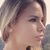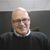# QlikView App Dev

Discussion Board for collaboration related to QlikView App Development.

Announcements
QlikWorld 2023, a live, in-person thrill ride. Save \$300 before February 6: REGISTER NOW!
cancel
Showing results for
Did you mean:Creator

## Help improve a query

Hi,

"internal inconsistency type a"

The amount of rows there is in ProductTemp QVD is: 363,704,000

Product:
DocNum,
InvoiceNum,
InvoiceLine,
ZAEHK,
WebCode,
TPLST,
DeliveryNum,
SUM(if(KSCHL = 'Z130'AND KINAK <> 'M',KWERT)) as Discount1,
SUM(if(KSCHL = 'ZCO2'AND KINAK <> 'M',KWERT)) as Discount2 ,
SUM(if(MATCH(KSCHL,'ZCO5','ZCO3'),KWERT)) as DiSountOrder1,
SUM(if(KSCHL = 'R100',KWERT)) as Discount100%,
SUM(if(MATCH(KSCHL,'PI02','ZPI2'),KWERT)) as Discount3,
SUM(if(MATCH(KSCHL,'PI01','ZPI1'),KWERT)) as FriendPrice,
SUM(if(KSCHL = 'ZCOS',KWERT)) as Discount4,
SUM(if(KSCHL = 'DIFF',KWERT)) as DiscountDiff,
SUM(if(MATCH(KSCHL,'ZBO3'),KWERT)) as Discount5,
SUM(if(KSCHL= 'ZE23',KWERT)) as Delivery,
SUM(if(KSCHL= 'ZE28',KWERT)) as Discount6,
SUM(if(KSCHL= 'ZE25',KWERT)) as Discount7,
SUM(if(KSCHL= 'ZE24',KWERT)) as Insurance,
SUM(if(KSCHL= 'ZE29',KWERT)) as Promotional,
SUM(if(KSCHL= 'ZV00',KWERT)) AS Product,
SUM(if(KSCHL= 'ZV10',KWERT)) AS Packaging,
SUM(if(KSCHL= 'ZV90',KWERT)) AS Contracting,
SUM(if(KSCHL= 'ZV15',KWERT)) AS Depreciation,
SUM(if(KSCHL= 'ZV60',KWERT)) AS Unfit,
SUM(if(KSCHL= 'ZV30',KWERT)) AS Permanentjob,
SUM(if(KSCHL= 'ZV05',KWERT)) AS Tempwork,
SUM(if(KSCHL= 'ZV40',KWERT)) AS MchinePermanent,
SUM(if(KSCHL= 'ZV45',KWERT)) AS TempMchine,
SUM(if(KSCHL= 'ZV70',KWERT)) AS TempDepreciation,
SUM(if(KSCHL= 'ZV80',KWERT)) AS Production,
SUM(if(KSCHL= 'Z120',KWERT)) AS Quantityofsalesinliters,
SUM(if(KSCHL= 'Z121',KWERT)) AS Quantityofsaleinkilograms,
SUM(if(KSCHL= 'Z110',KWERT)) AS QuantityofsaleinUnit,
SUM(if(KSCHL= 'Z100',KWERT)) AS Grosssales,
SUM(if(KSCHL= 'ZACR',KWERT)) AS Storage,
SUM(if([Material Type_MTART]='FERT' AND [Strategy group_STRGR]='82' and KSCHL= 'ZVPS',KWERT)) AS MixProduct,
SUM(if([Material Type_MTART]='FERT' AND [Strategy group_STRGR]='42' and KSCHL= 'ZVPS',KWERT)) AS MixCompany,
SUM(if(MATCH([Material Type_MTART],'PRFG','ROH','LABL','VERP','ZPRG') and KSCHL= 'ZVPS',KWERT)) AS TempProduct1,
SUM(if(KSCHL= 'ZPRS',KWERT)) AS Sellingcost

FROM ProductTemp.QVD (qvd)
Where KINAK <> 'Y'
GROUP BY DocNum,InvoiceNum,InvoiceLine,ZAEHK,WebCode,TPLST,DeliveryNum;

STORE Product into ProductFinal.QVD;

10 RepliesMVP

I assume, This is the way how it is in the rules. But, Can't you use them in design or calculate in Source side?

Before develop something, think If placed (The Right information | To the right people | At the Right time | In the Right place | With the Right context)Creator
Author

If I understood your question correctly,

There are a few more QVW that are using the "FinalProducd" QVD in other queriesCreator

How many rows of data do you get by just filtering on KINAK <> 'Y'?

How many rows of data did your original code get in the product table?Creator III

Hi Bellesol,

I think the many Sum(If...) aggregations in your load script are very resource consuming. Try to use flags, instead.

This example for the two fields Delivery and Discount6

T1:
DocNum,
InvoiceNum,
InvoiceLine,
ZAEHK,
WebCode,
TPLST,
DeliveryNum,
KSCHL,
KWERT,
If(KSCHL = 'ZE23', 1, 0) as Flag_Delivery,
If(KSCHL = 'ZE28', 1, 0) as Flag_Discount6,

.

.

.

FROM ProductTemp.QVD (qvd)
Where KINAK <> 'Y'
;

In the next step you can use the summing aggregation like this:

Sum(KWERT * Flag_Delivery) as Delivery

This will be loaded way faster...

Hope this helps

BurkhardCreator
Author

How many rows of data do you get by just filtering on KINAK <> 'Y' - 266,161,021

How many rows of data did your original code get in the product table - 266,371,372Creator
Author

HI,

The problem is that I need to sum the results,

for example:

SUM(if(KSCHL= 'ZE23',KWERT)) as Delivery,

I need to sum the result of "KWERT" - it can be 100,200

an example for the data inside the table:

 Material Type_MTART Strategy group_STRGR WebCode ZAEHK DocNum InvoiceNum DeliveryNum TPLST KSCHL KWERT InvoiceLine KINAK FERT 40 100 01 0015235795 0086325318 0000396278 1090 MWST 631.18 000020 X FERT 40 200 01 0015212322 0086320334 0000396288 1000 DIFF 100 000010 FERT 52 300 01 0015212322 0086320331 0000396222 1000 DIFF 200 000010Creator III

... of course, you need summing!

But this should be the second step after building the flags.

In a new resident load with the new created flag your summing statement looks like this:

Noconcatenate

T2:
DocNum,
InvoiceNum,
InvoiceLine,
ZAEHK,
WebCode,
TPLST,
DeliveryNum,
Sum(KWERT * Flag_Delivery) as Delivery,
.
.
.

Resident T1
Group By
DocNum,
InvoiceNum,
InvoiceLine,
ZAEHK,
WebCode,
TPLST;

Drop Table T1;

Happy qliking

BurkhardPartner - Champion III

The key to any task that is *heavy* is to follow a divide-and-conquer strategy.

So here is my first take on that:

1) Try to reduce the amount of data as much as possible from the outset - create the minimum viable subset of data you need for your analysis - by the most efficient way for your existing tool (QlikView LOAD statement in this case).

2) Consider the data model - do you need one single supertable as a result - you might not - as splitting the data between two or more tables might be more performant and efficient for QlikView.

STEP 1:

Do an "optimized load" first - then you can't create any new fields or use a regular WHERE statement except a WHERE EXISTS(...).

So that would mean:

``````COST_TYPE:
KSCHL, CostType
Z130, Discount1
ZC02, Discount2
ZC05, DiSountOrder1
ZC03, DiSountOrder1
HB00, DiscountSale2
.... fill in the remaining 28 or so rows with KSCHL values and their costttype
];

PRODUCT_DIMENSIONS_AND_KWERT:
Docnum,
InvoiceNum,
InvoiceLine,
ZAEHK,
WebCode,
TPLST,
DeliveryNum,
KSCHL,
[Material Type_MTART],
[Strategy group_STRGR],
KINAK,
KWERT
FROM
ProductTemp.QVD(qvd)
WHERE
Exists(KSCHL);
// I hope that this will have brought down the number of rows to a decent level

STORE PRODUCT_DIMENSIONS_AND_KWERT INTO Product_Dimensions_and_KWERT.QVD (qvd);

DROP TABLE PRODUCT_DIMENSIONS_AND_KWERT;

KINAK_FILTER:
KINAK
Y
];

PRODUCT_DIMENSION_AND_KWERT:
*
FROM
PRODUCT_DIMENSIONS_AND_KWERT FROM Product_Dimensions_and_KWERT.QVD (qvd)
WHERE
Not(Exists(KINAK));

STORE PRODUCT_DIMENSIONS_AND_KWERT INTO Product_Dimensions_and_KWERT.QVD (qvd);

// Now you have two tables, the COST_TYPE and PRODUCT_DIMENSIONS_AND_KWERT
// This might be a sufficent data model. However it can be further refined
// and it MIGHT give better performance by doing the following steps:

DROP TABLE PRODUCT_DIMENSIONS_AND_KWERT;

PRODUCT_DIMENSIONS:
Docnum,
InvoiceNum,
InvoiceLine,
ZAEHK,
WebCode,
TPLST,
DeliveryNum,
KSCHL,
[Material Type_MTART],
[Strategy group_STRGR],
AutoNumber(
Docnum & '|'
InvoiceNum & '|'
InvoiceLine & '|'
ZAEHK & '|'
WebCode & '|'
TPLST & '|'
DeliveryNum & '|'
KSCHL & '|'
[Material Type_MTART] & '|'
[Strategy group_STRGR],
'PROD_DIMS_KEY'
) AS %ProdDims
FROM
Product_Dimensions_and_KWERT.QVD (qvd);

METRICS:
AutoNumber(
Docnum & '|'
InvoiceNum & '|'
InvoiceLine & '|'
ZAEHK & '|'
WebCode & '|'
TPLST & '|'
DeliveryNum & '|'
KSCHL & '|'
[Material Type_MTART] & '|'
[Strategy group_STRGR],
'PROD_DIMS_KEY'
) AS %ProdDims,
KWERT
FROM
Product_Dimensions_and_KWERT.QVD (qvd);``````

In your UI you can calculate any particular cost type by having an expression like this using "Set Analysis" (it is really a set expression):

Sum( { <CostType={Discount1}> KWERT)

and in a Filter Pane your could also select the CostType more dynamically by just selecting which cost type to calculate.

Sum( {<CostType={MixProduct},[Material Type_MTART]={FERT},[Strategy group_STRGR]={82}>} KWERT)

In the case of the more composite classifications.

___________________________________________________________________

To make it even more "complete" and clean as a data model and include the composite classifications you could evolve the COST_TYPE table by following this approach:

You will have to refine the PRODUCT_TYPE table with three additional fields; [Material Type_MTART], [Strategy group_STRGR] and an AutoNumber([Mater...]&'|'&[Strat...],'PROD_TYPE_KEY') AS %ProdType (in both the COST_TYPE and PRODUCTION_DIMENSIONS table) and call the KSCHL field in the COST_TYPE table something like KSCHL_ (to avoid a synthetic key being generated by Qlik).

This is basically similar to the way that has already been done between the METRICS and PRODUCT_DIMENSIONS table...Creator

The "internal inconsistency type a" happens when the machine you are doing the reload on runs out of memory. The QMC publisher might have more memory than the machine you are trying to manually reload on. While the query can be optimized, it might still not run if your computer does not have enough memory.

There are many ways to improve the data model for performance if you consider how the result is used later on. Since you said the ProductFinal.QVD is used somewhere else and without knowing how it gets used, I can suggest just doing filter on KINAK here and IF(...,KWRT,0) without SUM() or Group By.

The Load script would then be:

PRODUCT:
DocNum,
InvoiceNum,
InvoiceLine,
ZAEHK,
WebCode,
TPLST,
DeliveryNum,
if(KSCHL = 'Z130'AND KINAK <> 'M',KWERT,0) as Discount1,
...
FROM ProductTemp.QVD (qvd)
Where KINAK <> 'Y'
STORE Product into ProductFinal.QVD;

Some explanations:
Group Bys are bottlenecked by a single threaded component - I think Qlik just sucks at doing this in general. It is not worth doing unless you expect to reduce the rows by more than 100x. Your data in and out of the group by has essentially the same number of rows, so it is useless.

Of course if you are allowed to change more downstream processes where the results are used, then you can use further optimization methods like others have suggested.Community Browser